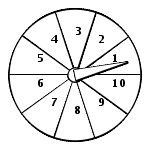# SHSAT Math

This is a demo for SHSAT Math but the questions are not necessarily suitable for you.

 What is the value of 2020200/20 ?
A) 1010100               B) 1110               C) 10101             D) 111                     E) 101010

 When expressing 1/11 as a decimal, you get 0.0909090909090909..., what is the 24th place to the right of the decimal point?
A) 8                      B) 2                          C) 9                          D) 4                              E) 0

 The following sequence of numbers are placed in the table as shown. Note that each number in the sequence is an even number. If the pattern continues, in which column will the number 372 appear?

 I II III IV V VI VII VIII IX X 0 2 4 6 8 10 12 14 16 18 20 22 24 26 28 30 32 34 36 38 40 42 ... ... ... ... ... ... ... ... ...

A) IV                 B) VII                      C) IX                         D) VI                            E) X

 Which of the following can NOT be the product of two consecutive odd numbers?
A) 260877               B) 682275             C) 12040899             D) 3599            E) 374543

 If X = 9/10 , Y = 10/11 and Z = 42/50 , which of the following is correct?
A) Z < X < Y        B) Z < Y < X        C) Y < Z < X         D) X < Y < Z         E) X < Z < Y

 Which of the following has the largest value?
A) √ 39 + √ 47     B) √ 42 + √ 44        C) √ 43 + √ 43    D) √ 43 + √ 43    E) √ 35 + √ 51

 P, Q, R,and S are on a number line with S not shown in the below graph. The length of RS is 4 times the length of PQ. What is a possible coordinate of point S?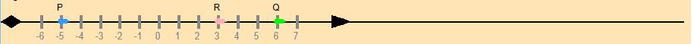A) 44          B) -42         C) 45            D) -41              E) -45

 The graph below shows the sales of various flavors in an ice cream shop. If 40 Coffee flavor ice creams were sold, what is the number of Chocolate flavor ice creams sold in the shop?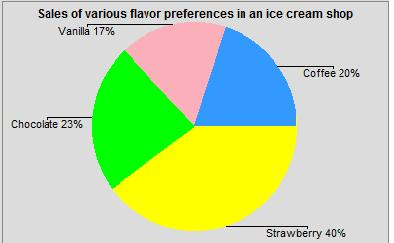A) 45            B) 43             C) 44                 D) 50                  E) 46

 If each of the fractions 2/X , 3/X , and 4/X is in its lowest terms, which of the following could be a value for X?
A) 45                   B) 30                   C) 64                     D) 31                  E) 60

 P and Q are natural numbers and P + Q = 15. What is the largest possible value of 1/P + 1/Q ?
A) 1+1/13           B) 1+ 1/14           C) 1+ 1/12        D) 1+ 1/2               E) 15/56

 All the three sides of a triangle have integer lengths. If one of the sides is 20, what is the smallest possible perimeter of the triangle?
A) 41             B) 38          C) 40           D) 43                 E) can not be determined

 If a@b means (a + b) /5 , then what will 25@(24@26) = ?
A) 4                 B) 3                C) 7                 D) 6                   E) 9

 If √ x + 1 /√ x = 4, then the value of 1 + x2 /x is
A) 14               B) 10             C) 11                  D) 13                   E) 12

 .(8) - .000(1) = ?
A) .8887                B) .79997                 C) .887               D) .0007               E) .88887

 ABCD is a rectangle. E, and F are at the same height. If the area of the rectangle ABCD is 54, what is the area sum of the shaded triangles?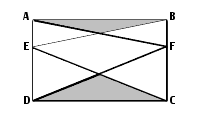A) 13.5            B) 11.5            C) 14.5             D) 10.5             E) can not be determined

 Two triangles ABC and BCD share a common side BC. Below are the angles in degrees.

 Angle BAC ABC BCA CDB DBC BCD Degrees(°) 54 62 64 66 55 59

Which of the following is the longest line segment? Note that the diagram might not be drawn to scale.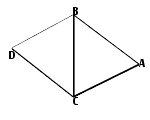A) BD                B) AB                C) CD                 D) AC                      E) BC

 In the figure below, three vertices A, B, and C of a quadrilateral fall on the circle circumference and the fourth at the circle center O. The measure of angle BCO = 67° and BAO = 68°. What is the measure of angle ABC?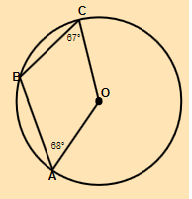A) 145°   B) 135°   C) 140°   D) 120°  E) It cannot be determined from the information given.

 Nick sells candy bars at a tailgate party to raise money for his school sports club. The candy bars are sold at 3 for \$2, and he brought them at 5 for \$1. How many candy bars must be sold in order to raise \$21?
A) 48                 B) 44                  C) 45                    D) 40                   E) 38

 ABCD is a rectangle and DEC is a triangle. If the degree measure of ADE : AED : BCE = 2 : 4 : 1, what is the degree measure of DEC? Note that the diagram might not be drawn to scale.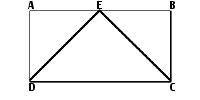A) 50         B) 45            C) 30            D) 35              E) can not be determined

 A spinner shown in the diagram has 10 equal sections. The probability of the pointer falling into each section is the same (Assume the pointer will never fall on a line between two sections). What is the possibility that the product of the two numbers that occur in two tries is a prime?